# Resources tagged with: GeoGebra

Filter by: Content type:
Age range:
Challenge level:

### There are 90 results

Broad Topics > Physical and Digital Manipulatives > GeoGebra### Square Coordinates

##### Age 11 to 14Challenge Level

A tilted square is a square with no horizontal sides. Can you devise a general instruction for the construction of a square when you are given just one of its sides?### A Tilted Square

##### Age 14 to 16Challenge Level

The opposite vertices of a square have coordinates (a,b) and (c,d). What are the coordinates of the other vertices?### Partitioning Revisited

##### Age 11 to 14Challenge Level

We can show that (x + 1)² = x² + 2x + 1 by considering the area of an (x + 1) by (x + 1) square. Show in a similar way that (x + 2)² = x² + 4x + 4### Overlap

##### Age 11 to 14Challenge Level

A red square and a blue square overlap so that the corner of the red square rests on the centre of the blue square. Show that, whatever the orientation of the red square, it covers a quarter of the. . . .### Colour in the Square

##### Age 7 to 16Challenge Level

Can you put the 25 coloured tiles into the 5 x 5 square so that no column, no row and no diagonal line have tiles of the same colour in them?### Farey Sequences

##### Age 11 to 14Challenge Level

There are lots of ideas to explore in these sequences of ordered fractions.### Shear Magic

##### Age 11 to 14Challenge Level

Explore the area of families of parallelograms and triangles. Can you find rules to work out the areas?### L-triominoes

##### Age 14 to 16Challenge Level

L triominoes can fit together to make larger versions of themselves. Is every size possible to make in this way?### Mediant Madness

##### Age 14 to 16Challenge Level

Kyle and his teacher disagree about his test score - who is right?### More Twisting and Turning

##### Age 11 to 16Challenge Level

It would be nice to have a strategy for disentangling any tangled ropes...### Quad in Quad

##### Age 14 to 16Challenge Level

Join the midpoints of a quadrilateral to get a new quadrilateral. What is special about it?### Beelines

##### Age 14 to 16Challenge Level

Is there a relationship between the coordinates of the endpoints of a line and the number of grid squares it crosses?### Tilting Triangles

##### Age 14 to 16Challenge Level

A right-angled isosceles triangle is rotated about the centre point of a square. What can you say about the area of the part of the square covered by the triangle as it rotates?### Same Length

##### Age 11 to 16Challenge Level

Construct two equilateral triangles on a straight line. There are two lengths that look the same - can you prove it?### The Medieval Octagon

##### Age 14 to 16Challenge Level

Medieval stonemasons used a method to construct octagons using ruler and compasses... Is the octagon regular? Proof please.### Picturing Triangular Numbers

##### Age 11 to 14Challenge Level

Triangular numbers can be represented by a triangular array of squares. What do you notice about the sum of identical triangle numbers?### Cyclic Quadrilaterals

##### Age 11 to 16Challenge Level

Draw some quadrilaterals on a 9-point circle and work out the angles. Is there a theorem?### More Magic Potting Sheds

##### Age 11 to 14Challenge Level

The number of plants in Mr McGregor's magic potting shed increases overnight. He'd like to put the same number of plants in each of his gardens, planting one garden each day. How can he do it?### Arrowhead

##### Age 14 to 16Challenge Level

The points P, Q, R and S are the midpoints of the edges of a non-convex quadrilateral.What do you notice about the quadrilateral PQRS and its area?### Is There a Theorem?

##### Age 11 to 14Challenge Level

Draw a square. A second square of the same size slides around the first always maintaining contact and keeping the same orientation. How far does the dot travel?### Pythagoras Proofs

##### Age 14 to 16Challenge Level

Can you make sense of these three proofs of Pythagoras' Theorem?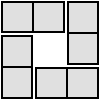### 4 Dom

##### Age 5 to 16Challenge Level

Use these four dominoes to make a square that has the same number of dots on each side.### Just Rolling Round

##### Age 14 to 16Challenge Level

P is a point on the circumference of a circle radius r which rolls, without slipping, inside a circle of radius 2r. What is the locus of P?### Guesswork

##### Age 14 to 16Challenge Level

Ask a friend to choose a number between 1 and 63. By identifying which of the six cards contains the number they are thinking of it is easy to tell them what the number is.### Squaring the Circle and Circling the Square

##### Age 14 to 16Challenge Level

If you continue the pattern, can you predict what each of the following areas will be? Try to explain your prediction.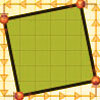### At Right Angles

##### Age 14 to 16Challenge Level

Can you decide whether two lines are perpendicular or not? Can you do this without drawing them?### Factor Lines

##### Age 7 to 14Challenge Level

Arrange the four number cards on the grid, according to the rules, to make a diagonal, vertical or horizontal line.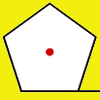### How Far Does it Move?

##### Age 11 to 14Challenge Level

Experiment with the interactivity of "rolling" regular polygons, and explore how the different positions of the red dot affects the distance it travels at each stage.### Rolling Around

##### Age 11 to 14Challenge Level

A circle rolls around the outside edge of a square so that its circumference always touches the edge of the square. Can you describe the locus of the centre of the circle?### The Farmers' Field Boundary

##### Age 11 to 14Challenge Level

The farmers want to redraw their field boundary but keep the area the same. Can you advise them?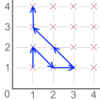### Areas from Vectors

##### Age 11 to 16

Use the applet to explore the area of a parallelogram and how it relates to vectors.### Teddy Town

##### Age 5 to 14Challenge Level

There are nine teddies in Teddy Town - three red, three blue and three yellow. There are also nine houses, three of each colour. Can you put them on the map of Teddy Town according to the rules?### Triangle in a Triangle

##### Age 14 to 16Challenge Level

Can you work out the fraction of the original triangle that is covered by the inner triangle?### Triangles in Circles

##### Age 11 to 14Challenge Level

Can you find triangles on a 9-point circle? Can you work out their angles?### Coordinates of Corners

##### Age 11 to 16

Use the applet to make some squares. What patterns do you notice in the coordinates?### Right Angles

##### Age 11 to 14Challenge Level

Can you make a right-angled triangle on this peg-board by joining up three points round the edge?### Magic Potting Sheds

##### Age 11 to 14Challenge Level

Mr McGregor has a magic potting shed. Overnight, the number of plants in it doubles. He'd like to put the same number of plants in each of three gardens, planting one garden each day. Can he do it?### Napoleon's Theorem

##### Age 14 to 18Challenge Level

Triangle ABC has equilateral triangles drawn on its edges. Points P, Q and R are the centres of the equilateral triangles. What can you prove about the triangle PQR?### Tessellation Interactivity

##### Age 7 to 16Challenge Level

An environment that enables you to investigate tessellations of regular polygons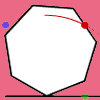### Up and Across

##### Age 11 to 14Challenge Level

Experiment with the interactivity of "rolling" regular polygons, and explore how the different positions of the dot affects its vertical and horizontal movement at each stage.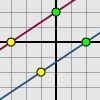### Translating Lines

##### Age 11 to 14Challenge Level

Investigate what happens to the equation of different lines when you translate them. Try to predict what will happen. Explain your findings.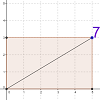### Exploring Diagonals

##### Age 11 to 16

Move the corner of the rectangle. Can you work out what the purple number represents?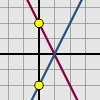### Reflecting Lines

##### Age 11 to 14Challenge Level

Investigate what happens to the equations of different lines when you reflect them in one of the axes. Try to predict what will happen. Explain your findings.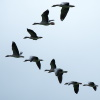### Solving Together - Estimating Angles

##### Age 11 to 14

Week 2
How well can you estimate angles? Playing this game could improve your skills.### Subtended Angles

##### Age 11 to 14Challenge Level

What is the relationship between the angle at the centre and the angles at the circumference, for angles which stand on the same arc? Can you prove it?### Reflecting Squarely

##### Age 11 to 14Challenge Level

In how many ways can you fit all three pieces together to make shapes with line symmetry?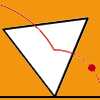### Speeding Up, Slowing Down

##### Age 11 to 14Challenge Level

Experiment with the interactivity of "rolling" regular polygons, and explore how the different positions of the red dot affects its speed at each stage.### Rollin' Rollin' Rollin'

##### Age 11 to 14Challenge Level

Two circles of equal radius touch at P. One circle is fixed whilst the other moves, rolling without slipping, all the way round. How many times does the moving coin revolve before returning to P?### Bow Tie

##### Age 11 to 14Challenge Level

Show how this pentagonal tile can be used to tile the plane and describe the transformations which map this pentagon to its images in the tiling.### Isosceles Triangles

##### Age 11 to 14Challenge Level

Draw some isosceles triangles with an area of $9$cm$^2$ and a vertex at (20,20). If all the vertices must have whole number coordinates, how many is it possible to draw?